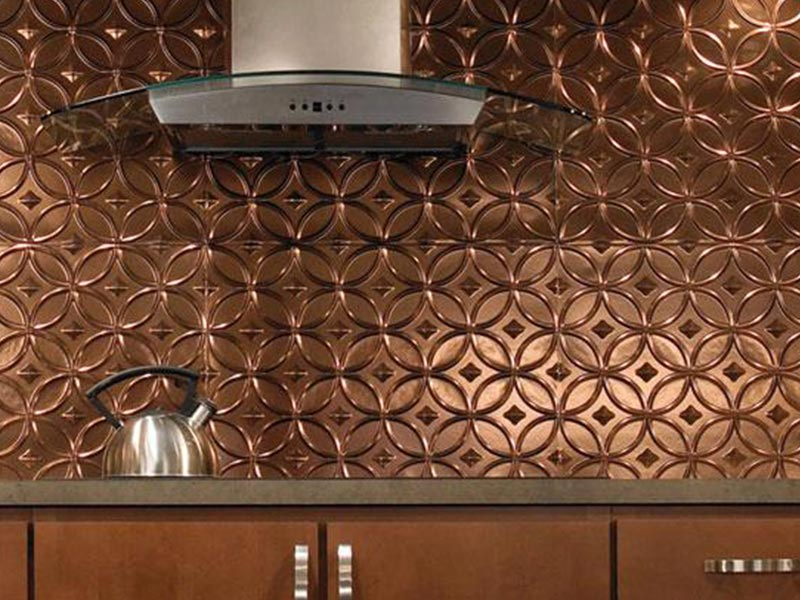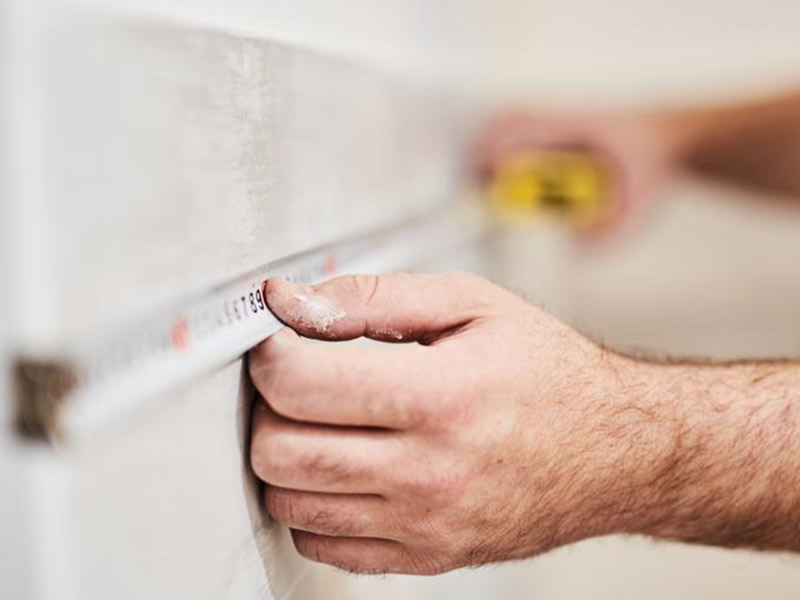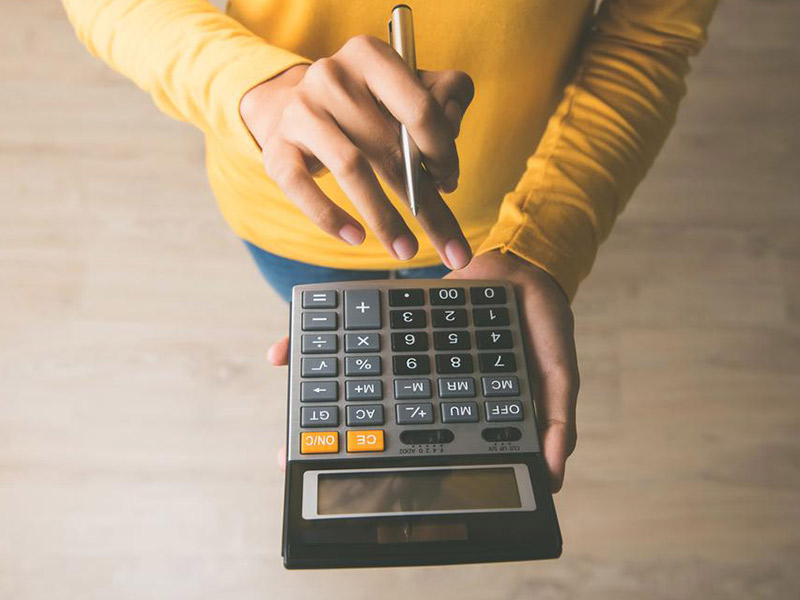# How to Measure for Your Tile Backsplash Area

Written by Milan Jara on 26th Jul 2022Confused as to how to measure for tile backsplash? We provide tips and techniques to determine the precise measurement and how much you will need.

The important first step to beginning a tiling installation is knowing how many tiles to order. You are not simply calculating square footage but how much extra you will require according to the material. Ordering a bit extra is always a wise idea since you may make mistakes. We teach you how to measure for tile backsplash and calculate what you will need.

## How to Measure Tile BacksplashThe basic measurement for calculating square feet is done using the following equation:

Length (in inches) X Width (in inches) = Total area squared (in inches)

To calculate square footage, you then use the following equation:

Total square inch area/144 = Total square footage

These equations are relatively simple and appear straightforward on the surface. However, there is one tiny problem, and that is that many areas are not a perfect rectangle. This is where all the math you learned in school (and a tape measure) comes in handy.

### Irregularly Shaped areas

For rooms that are irregularly shaped or for irregular spaces, like when doing a kitchen backsplash, you will need to calculate the square footage by breaking the area into smaller rectangles to generate an accurate measurement.

To do this, label each area with a sticky note or piece of tape. You can label them in numbers, letters, or anything you choose. This is done so that you can differentiate the different areas both before installation and while you are installing the tile backsplash.

Areas should be marked off in as few rectangles as possible. It also helps to draw out your area using a piece of paper, which will allow you to keep track of each number.

Then, use a measuring tape to measure each section, record it, and calculate your square footage for each section using the formula above. After that is done, add each section together to get your total.

Measurements don’t have to be incredibly precise since you will order 10-20% over your total. You’ll need this extra in case any of the tiles break and to account for installation waste. It is always best to have more than not enough. Also, always round your total up to the nearest whole number, never down.

### Calculating Backsplash TileIn addition to the total surface area, you will need to know how many tiles you need to cover it. It is easy to convert your coverage area into a tile count.

To do this, you will have to find the area of a single tile. All you need to do is multiply length times width in inches. Once you have your calculation, divide the area of the space by the tile’s area. This will indicate how many tiles are required to complete the project.

Example:

Each individual tile measures 24” x 24”, indicating that one pack will cover 9.7 square feet. If you have tiles that are 24” x 24”, and your area is 48” in width by 24” in height:

• Start by calculating the total area of an individual tile: 24 x 24 = 576”
• Determine the area of your backsplash: 1,152 square inches
• Divide the space by a single wall tile: 1,152/576 is two tiles that you will need to complete the project.

### Calculating Waste

Each project has some degree of waste since tiles will require cutting and some may break. Purchase an additional 10 to 15% in extra square feet to account for waste. You may need to go as high as 20%, depending on the project and the tile.

To calculate how much extra to order according to material, the following can be used as a guideline:

• Irregular shaped rooms: 15-20% as there will be corners that require cutting
• Detailed designs and patterns: 15-20%
• Natural stone: 10-15% in waste as this material has the potential to break easily during the tile installation
• Ceramics and porcelain: 10% waste

To calculate the total amount you will require, multiply the square footage by 1.10 for a 10% waste, 1.15 for a 15% waste, and 1.20 for a 20% waste.

### Other Tips

One shortcut you can do if you have a window that separates two areas is to completely measure the windowed area (length X width divided by 144 inches). Then, measure the area of the window. Take the window measurement and subtract it from the total area for your square footage.

Also, the grout line area is considered cutting waste and is not added to your calculation. Backsplashes using diagonal cuts should contain a waste allowance of a minimum of 10%. Since these tiles come in dye lots and batches, extra tile will need to be purchased and stored in a dry area. This is if you need to replace any of your tiles in the future.

• If measuring for a shower, consider benches and niches in your calculations. If installing a border tile, measure the perimeter and divide by 12 for the total linear feet.
• For measuring a fireplace, consider your mantel’s width plus overhang. Measure the height from your floor to the top of your opening. For square footage, multiply your width by your height.
• Regarding walls, calculate the area of the complete wall. Subtract the area from windows or other elements on your wall from the total.

In how to measure for tile backsplash, you will need to consider the tile size, layout, shape, and overage. All these calculations come into play when determining the square footage required for your project.

Buy Ceiling Tiles in Bulk & Get 10% - 15% off + Free Shipping

Buy Ceiling Tiles in Bulk & Get 10% - 15% off + Free Shipping